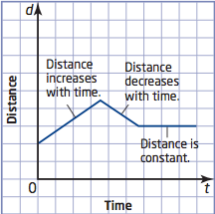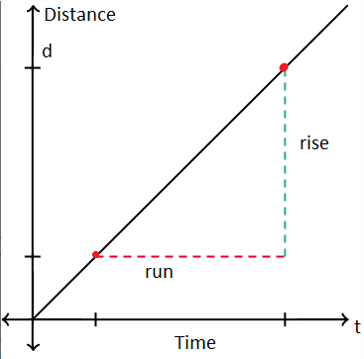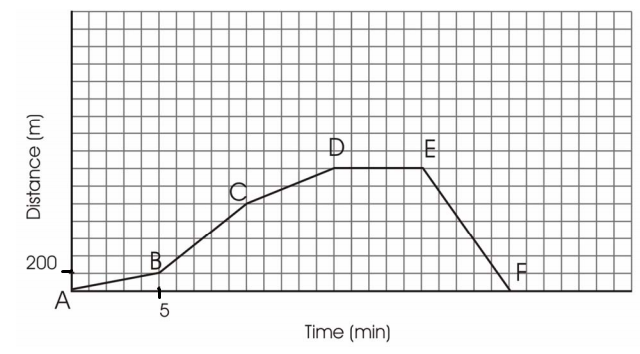# Distance vs Time Graphs

One of the most common examples of a linear relation is the relationship between distance and time when a travelling at a constant speed. For exmaple, if you are travelling 40 km/h then every hour you will travel 40 km.

A distance-time graph shows an object's distance from a fixed point over a period of time.A rising line shows that the object's distance increases away from the reference point.

A falling line shows that the object's distance decreases towards the reference point.

A flat line shows the object isn't moving!

## Velocity

In distance vs time graphs, the distance should be represented on the vertical axis. Time would be the horizontal axis, and the velocity would be represented by the slope of the graph.

In order to calculate the slope, we can use the equation:

$$m = \cfrac{rise}{run} = \cfrac{y_2-y_1}{x_2-x_1} = \cfrac{d_2-d_1}{t_2-t_1} = v$$

From this equation we can see that the speed is distance over time:

$$v = \cfrac{d}{t}$$## D vs T Graphs

To better demonstrate how to calculate rate of change on a distance-time graph refer to Timmy's shopping trip below.Try and calculate the speed at each segment of Timm'y trip, when was he moving the fastest?